## If your problem can be expressed in terms of convolutions and inner products then take the Fourier transform

### Quick description

There are many problems in mathematics that do not at first seem to concern the Fourier transform, but for which the Fourier transform can be a major help in the solution. This article is about how to recognise such problems.

### Prerequisites

A basic knowledge of the Fourier transform. (See for example the definitions and basic facts laid out in the Fourier transforms front page, and some of the further reading suggested there.)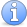Incomplete This article is incomplete. Many more examples needed. Perhaps the rest of the proof of Roth's theorem should be sketched?

### Example 1

Roth's theorem about arithmetic progressions is the following beautiful statement.

Theorem 1 For every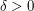there exists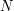such that every subset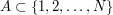of size at least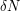contains an arithmetic progression of length 3.

One of the first ideas towards a proof is to come up with an analytic expression for the number of arithmetic progressions of length 3. (This is an instance of the idea of turning sets into functions.) We define the characteristic function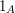of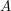by setting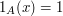if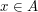andotherwise. Then the number of progressions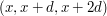with all three terms inis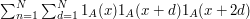.

We can make this expression a lot tidier and easier to analyse if we think of the numbers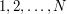as elements of the group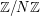. Then we can think about the expression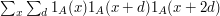, where bothand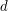range over all of. This is not equal to the previous expression, but the extra convenience of this definition more than compensates for the small technicalities needed to deal with the differences between it and the previous one.

Now let us rearrange this second expression as follows.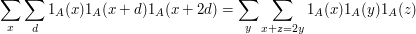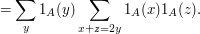This is, up to a normalizing factor, the inner product of a function very closely related towith the convolution ofwith itself. As such, we know it will have some simple expression in terms of the Fourier transform of.

Before we go ahead and give this expression, let us switch to a more standard normalization. We shall write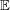for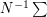, and instead of looking at the sum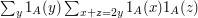we shall look at the expectation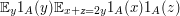. Also, we shall define the convolution of two functions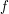and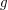fromto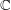by the formula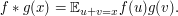Then we can rewriteas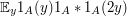. Ifis odd, then we can substitute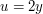and replace this by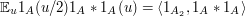, where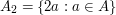.

We have now expressed our problem, or at least a quantity closely associated with our problem, in terms of convolutions and inner products. At this point we use Parseval's identity and the convolution identity to rewrite the expression as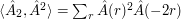. (See the Fourier transforms front page for a definition of the discrete Fourier transform and a discussion of these two identities.)

Why are we any better off? The reason is that now we have an unrestricted sum over one variable rather than a sum over three variables that satisfy an additive condition. Therefore, the sum we need to analyse can be estimated using more standard inequalities. This does not solve the problem, but it does turn out to be a very useful step in Roth's proof of his theorem, and in many other arguments of a similar nature.